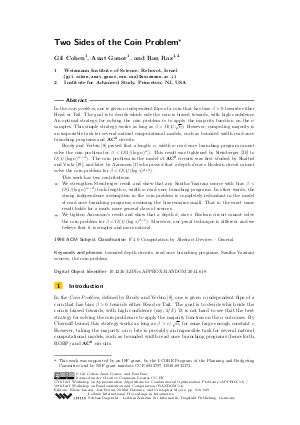Document# Two Sides of the Coin Problem

### Authors Gil Cohen, Anat Ganor, Ran Raz## File

LIPIcs.APPROX-RANDOM.2014.618.pdf
• Filesize: 495 kB
• 12 pages

## Cite As

Gil Cohen, Anat Ganor, and Ran Raz. Two Sides of the Coin Problem. In Approximation, Randomization, and Combinatorial Optimization. Algorithms and Techniques (APPROX/RANDOM 2014). Leibniz International Proceedings in Informatics (LIPIcs), Volume 28, pp. 618-629, Schloss Dagstuhl - Leibniz-Zentrum für Informatik (2014)
https://doi.org/10.4230/LIPIcs.APPROX-RANDOM.2014.618

## Abstract

In the coin problem, one is given n independent flips of a coin that has bias b > 0 towards either Head or Tail. The goal is to decide which side the coin is biased towards, with high confidence. An optimal strategy for solving the coin problem is to apply the majority function on the n samples. This simple strategy works as long as b > c(1/sqrt n) for some constant c. However, computing majority is an impossible task for several natural computational models, such as bounded width read once branching programs and AC^0 circuits. Brody and Verbin proved that a length n, width w read once branching program cannot solve the coin problem for b < O(1/(log n)^w). This result was tightened by Steinberger to O(1/(log n)^(w-2)). The coin problem in the model of AC^0 circuits was first studied by Shaltiel and Viola, and later by Aaronson who proved that a depth d size s Boolean circuit cannot solve the coin problem for b < O(1/(log s)^(d+2)). This work has two contributions: 1. We strengthen Steinberger's result and show that any Santha-Vazirani source with bias b < O(1/(log n)^(w-2)) fools length n, width w read once branching programs. In other words, the strong independence assumption in the coin problem is completely redundant in the model of read once branching programs, assuming the bias remains small. That is, the exact same result holds for a much more general class of sources. 2. We tighten Aaronson's result and show that a depth d, size s Boolean circuit cannot solve the coin problem for b < O(1/(log s)^(d-1)). Moreover, our proof technique is different and we believe that it is simpler and more natural.
##### Keywords
• bounded depth circuits
• Santha-Vazirani sources
• the coin problem

## Metrics

• Access Statistics
• Total Accesses (updated on a weekly basis)
0